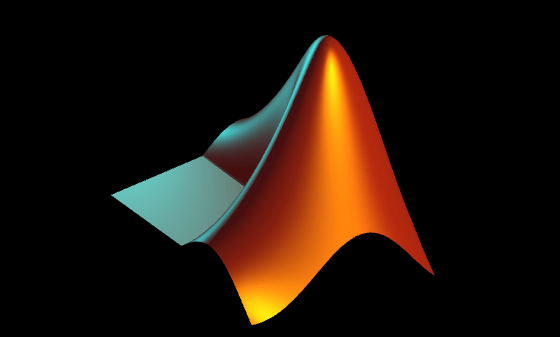# Creating the MATLAB Logo

This example shows how to create and display the MATLAB® logo.

Use the `membrane` command to generate the surface data for the logo.

`L = 160*membrane(1,100);`

Create a figure and an axes to display the logo. Then, create a surface for the logo using the points from the `membrane` command. Turn off the lines in the surface.

```f = figure; ax = axes; s = surface(L); s.EdgeColor = 'none'; view(3)```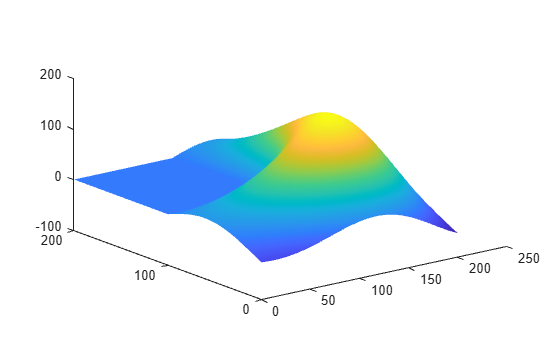Adjust the axes limits so that the axes are tight around the logo.

```ax.XLim = [1 201]; ax.YLim = [1 201]; ax.ZLim = [-53.4 160];```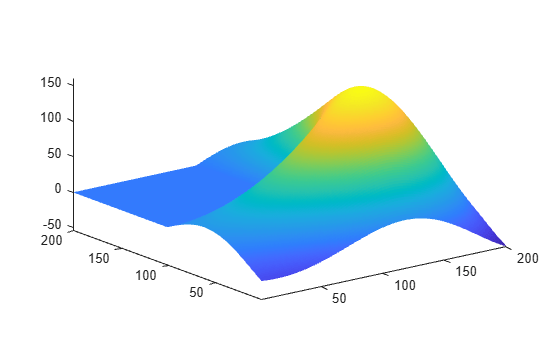Adjust the view of the logo using the camera properties of the axes. Camera properties control the view of a three dimensional scene like a camera with a zoom lens.

```ax.CameraPosition = [-145.5 -229.7 283.6]; ax.CameraTarget = [77.4 60.2 63.9]; ax.CameraUpVector = [0 0 1]; ax.CameraViewAngle = 36.7;```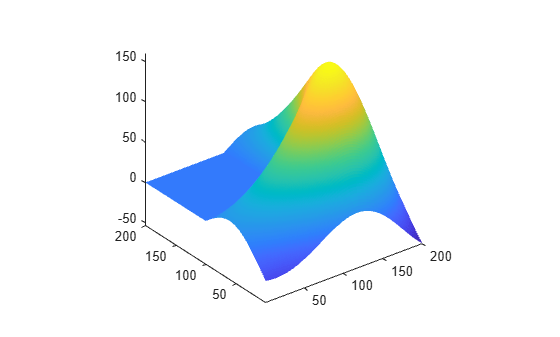Change the position of the axes and the x, y, and z aspect ratio to fill the extra space in the figure window.

```ax.Position = [0 0 1 1]; ax.DataAspectRatio = [1 1 .9];```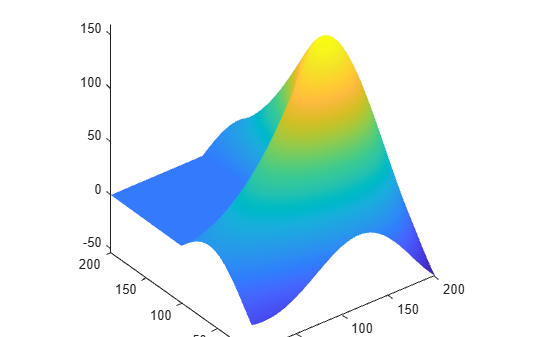Create lights to illuminate the logo. The light itself is not visible but its properties can be set to change the appearance of any patch or surface object in the axes.

```l1 = light; l1.Position = [160 400 80]; l1.Style = 'local'; l1.Color = [0 0.8 0.8]; l2 = light; l2.Position = [.5 -1 .4]; l2.Color = [0.8 0.8 0];```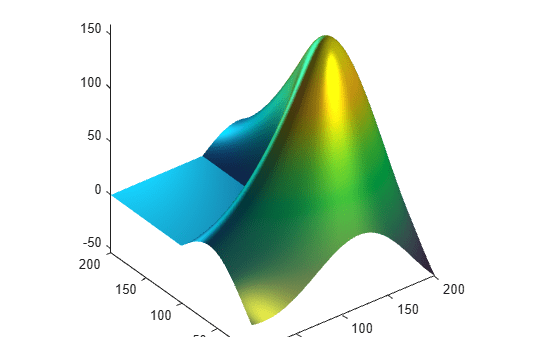Change the color of the logo.

`s.FaceColor = [0.9 0.2 0.2];`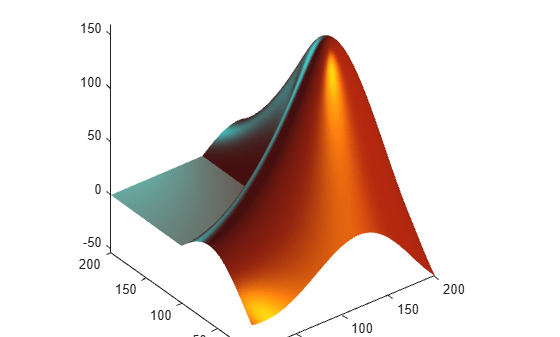Use the lighting and specular (reflectance) properties of the surface to control the lighting effects.

```s.FaceLighting = 'gouraud'; s.AmbientStrength = 0.3; s.DiffuseStrength = 0.6; s.BackFaceLighting = 'lit'; s.SpecularStrength = 1; s.SpecularColorReflectance = 1; s.SpecularExponent = 7;```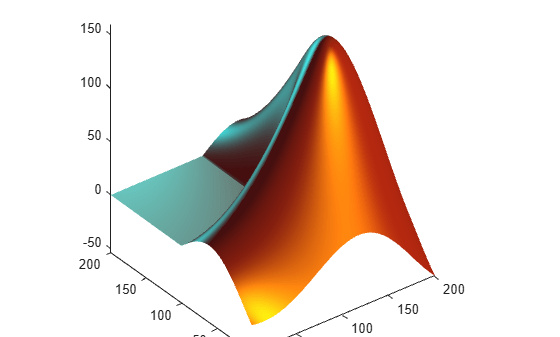Turn the axis off to see the final result.

```axis off f.Color = 'black';```